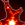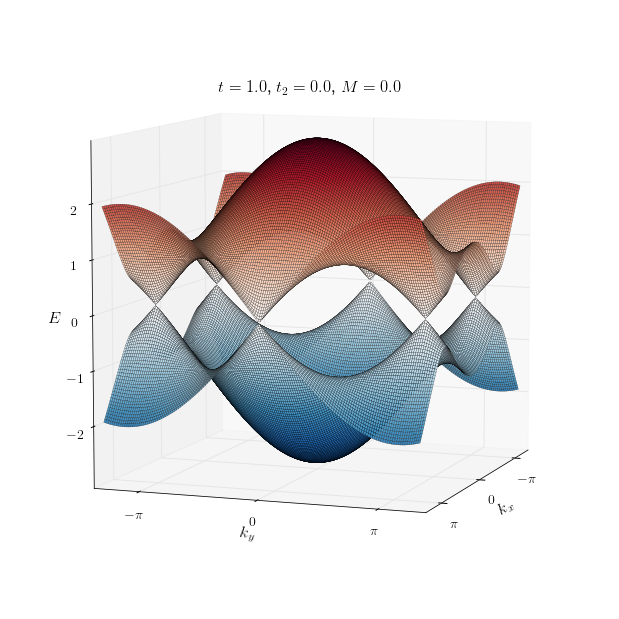CoCalc Public Filesw4_haldane / haldane_model.ipynb
Compute Environment: Ubuntu 18.04 (Deprecated)
In :
import sys
sys.path.append('../code')
from init_mooc_nb import *
init_notebook()
import scipy
from wraparound import wraparound
%output size=150
pi_ticks = [(-np.pi, r'$-\pi$'), (0, '0'), (np.pi, r'$\pi$')]

def haldane(w=20, boundary='zigzag'):
def ribbon_shape_zigzag(pos):
return (-0.5 / np.sqrt(3) - 0.1 <= pos < np.sqrt(3) * w / 2 + 0.01)

def ribbon_shape_armchair(pos):
return (-1 <= pos < w)

def onsite(site, p):
if site.family == a:
return p.m
else:
return -p.m

def nn_hopping(site1, site2, p):
return p.t

def nnn_hopping(site1, site2, p):
return 1j * p.t_2

lat = kwant.lattice.honeycomb()
a, b = lat.sublattices
nnn_hoppings_a = (((-1, 0), a, a), ((0, 1), a, a), ((1, -1), a, a))
nnn_hoppings_b = (((1, 0), b, b), ((0, -1), b, b), ((-1, 1), b, b))

if boundary == 'zigzag':
sys = kwant.Builder(kwant.TranslationalSymmetry((1, 0)))
sys[lat.shape(ribbon_shape_zigzag, (0, 0))] = onsite
elif boundary == 'armchair':
sys = kwant.Builder(kwant.TranslationalSymmetry((0, np.sqrt(3))))
sys[lat.shape(ribbon_shape_armchair, (0, 0))] = onsite
else:
sys = kwant.Builder(kwant.TranslationalSymmetry(*lat.prim_vecs))
sys[lat.shape(lambda pos: True, (0, 0))] = onsite

sys[lat.neighbors()] = nn_hopping
sys[[kwant.builder.HoppingKind(*hopping) for hopping in nnn_hoppings_a]] = nnn_hopping
sys[[kwant.builder.HoppingKind(*hopping) for hopping in nnn_hoppings_b]] = nnn_hopping

return sys

def Qi_Wu_Zhang():
def onsite(site, p):
return - p.mu * pauli.sz

def hopx(site1, site2, p):
return - 0.5j * p.delta * pauli.sy - p.t * pauli.sz

def hopy(site1, site2, p):
return - 1j * p.gamma * pauli.sx  - p.gamma * pauli.sz

lat = kwant.lattice.square()

sys = kwant.Builder(kwant.TranslationalSymmetry(*lat.prim_vecs))
sys[lat.shape(lambda pos: True, (0, 0))] = onsite
sys[kwant.HoppingKind((1,0), lat)] = hopx
sys[kwant.HoppingKind((0,1), lat)] = hopy
return sys

def berry_curvature(sys, p, ks, num_filled_bands=1):
"""Berry curvature of a system.

Parameters:
-----------
sys : kwant.Builder
A 2D infinite system.
p : SimpleNamespace
The arguments expected by the system.
ks : 1D array-like
Values of momentum grid to be used for Berry curvature calculation.
num_filled_bands : int
The number of filled bands.

Returns:
--------
bc : 2D array
Berry curvature on each square in a ks x ks grid.
"""
# Calculate an array of eigenvectors.
B = np.array(sys.symmetry.periods).T
A = B.dot(np.linalg.inv(B.T.dot(B)))

sys = wraparound(sys).finalized()

def energy(kx, ky):
k = np.array([kx, ky])
kx, ky = np.linalg.solve(A, k)
H = sys.hamiltonian_submatrix([p, kx, ky], sparse=False)
return scipy.linalg.eigh(H)

vectors = np.array([[energy(kx, ky)[:, :num_filled_bands] for kx in ks] for ky in ks])

# The actual Berry curvature calculation
vectors_x = np.roll(vectors, 1, 0)
vectors_xy = np.roll(vectors_x, 1, 1)
vectors_y = np.roll(vectors, 1, 1)

shifted_vecs = [vectors, vectors_x, vectors_xy, vectors_y]

v_shape = vectors.shape

shifted_vecs = [i.reshape(-1, v_shape[-2], v_shape[-1]) for i in shifted_vecs]

dets = np.ones(len(shifted_vecs), dtype=complex)
for vec, shifted in zip(shifted_vecs, np.roll(shifted_vecs, 1, 0)):
dets *= [np.linalg.det(a.T.conj().dot(b)) for a, b in zip(vec, shifted)]
bc = np.angle(dets).reshape(int(np.sqrt(len(dets))), -1)

bc = (bc + np.pi / 2) % (np.pi) - np.pi / 2

return bc

def plot_berry_curvature(sys, p, ks=None, title=None):
if ks is None:
ks = np.linspace(-np.pi, np.pi, 150, endpoint=False)
bc = berry_curvature(sys, p, ks)[1:-1,1:-1]
vmax = max(np.abs(bc).min(), np.abs(bc).max())
kwargs = {'bounds': (ks.min(), ks.min(), ks.max(), ks.max()),
'kdims': [r'$k_x$', r'$k_y$']}

if callable(title):
kwargs['label'] = title(p)

plot = {'xticks': pi_ticks, 'yticks': pi_ticks}
style = {'clims': [-vmax, vmax]}
return holoviews.Image(bc, **kwargs)(plot=plot, style=style)

def title(p):
title = r'$t={:.2}$, $t_2={:.2}$, $M={:.2}$'
return title.format(p.t, p.t_2, p.m)

Populated the namespace with: np, matplotlib, kwant, holoviews, init_notebook, interact, display_html, plt, pf, SimpleNamespace, pprint_matrix, scientific_number, pretty_fmt_complex from code/edx_components: MoocVideo, PreprintReference, MoocDiscussion, MoocCheckboxesAssessment, MoocMultipleChoiceAssessment, MoocPeerAssessment, MoocSelfAssessment from code/functions: spectrum, hamiltonian_array, h_k, pauliHoloViewsJS, MatplotlibJS successfully loaded in this cell.

Press this button to show/hide the code used in the notebook:

# Intro

Duncan Haldane from Princeton University will teach us about an interesting two dimensional toy-model which he introduced in 1988, and which has become a prototype for the anomalous quantum Hall effect.

In :
MoocVideo("7nVO4uMm-do", src_location='4.2-intro')We will now study the model in detail, starting from the beginning. Along the way, we will also learn about the Chern number, the bulk topological invariant of a quantum Hall state.

# Dirac cones in graphene

In the last chapter we saw how it is possible to obtain a quantum Hall state by coupling one-dimensional systems. At the end, our recipe was to first obtain a Dirac cone, add a mass term to it and finally to make this mass change sign. Following this recipe we were able to obtain chiral edge states without applying an external magnetic field.

There is a real (and a very important) two-dimensional system which has Dirac cones: graphene. So in this chapter we will take graphene and make it into a topological system with chiral edge states.

Graphene is a single layer of carbon atoms arranged in a honeycomb lattice. It is a triangular lattice with two atoms per unit cell, type $A$ and type $B$, represented by red and blue sites in the figure:Hence, the wave function in a unit cell can be written as a vector $(\Psi_A, \Psi_B)^T$ of amplitudes on the two sites $A$ and $B$. Taking a simple tight-binding model where electrons can hop between neighboring sites with hopping strength $t$, one obtains the Bloch Hamiltonian:

$H_0(\mathbf{k})= \begin{pmatrix} 0 & h(\mathbf{k}) \\ h^\dagger(\mathbf{k}) & 0 \end{pmatrix}\,,$

with $\mathbf{k}=(k_x, k_y)$ and

$h(\mathbf{k}) = t_1\,\sum_i\,\exp\,\left(i\,\mathbf{k}\cdot\mathbf{a}_i\right)\,.$

Here $\mathbf{a}_i$ are the three vectors in the figure, connecting nearest neighbors of the lattice [we set the lattice spacing to one, so that for instance $\mathbf{a}_1=(1,0)$]. Introducing a set of Pauli matrices $\sigma$ which act on the sublattice degree of freedom, we can write the Hamiltonian in a compact form as

$H_0(\mathbf{k}) = t_1\,\sum_i\,\left[\sigma_x\,\cos(\mathbf{k}\cdot\mathbf{a}_i)-\sigma_y \,\sin(\mathbf{k}\cdot\mathbf{a}_i)\right]\,.$

The energy spectrum $E(\mathbf{k}) = \pm \,\left|h(\mathbf{k})\right|$ gives rise to the famous band structure of graphene, with the two bands touching at the six corners of the Brillouin zone:

In :
p = SimpleNamespace(t=1.0, t_2=0.0, m=0.0, phi=np.pi/2)
sys = haldane(boundary='infinite')
k = (4 / 3) * np.linspace(-np.pi, np.pi, 150)
spectrum(sys, p, k_x=k, k_y=k, title=title)Only two of these six Dirac cones are really distinct, the ones at $\mathbf{K}=(2\pi/3, 2\pi/3\sqrt{3})$ and $\mathbf{K}'=(2\pi/3, -2\pi/3\sqrt{3})$. All the others can be obtained by adding some reciprocal lattice vector to $\mathbf{K}$ and $\mathbf{K}'$.

# Discrete symmetries of graphene

The symmetries of graphene were discussed intensively in the video, so let's review them.

As we already said in our first week, graphene is the prototype of a system with sublattice symmetry, which makes the Hamiltonian block off-diagonal with respect to the two sublattices. The sublattice symmetry reads

$\sigma_z\,H_0(\mathbf{k})\,\sigma_z = -H_0(\mathbf{k})\,.$

Sublattice symmetry is only approximate, and it is consequence of the nearest neighbor tight-binding model. Just like the inversion symmetry mentioned in the video, it protects the Dirac points and needs to be broken in order to open a gap.

In addition to sublattice and inversion symmetry, the honeycomb lattice also has a three-fold rotation symmetry around the center of the unit cell. This symmetry is important to make the Dirac cones appear in the first place, but it will not play a role in all that follows.

Finally, there is time-reversal symmetry, which at the moment is perfectly preserved in our tight-binding model. Since we are not considering the spin degree of freedom of the electrons, the time-reversal symmetry operator in real space is just complex conjugation. In momentum space representation, time-reversal symmetry reads

$H_0(\mathbf{k}) = H_0^*(-\mathbf{k})\,.$

It's important to note that time-reversal symmetry sends $\mathbf{K}$ into $\mathbf{K}'$ and therefore it exchanges the two Dirac cones.

The product of (approximate) sublattice and time-reversal symmetries yields a further discrete symmetry, a particle-hole symmetry $\sigma_z H^*(-\mathbf{k})\,\sigma_z = -H_0(\mathbf{k})$.

# Making graphene topological

Let's recall that our goal is to make our graphene sheet enter a quantum Hall state, with chiral edge states. The first necessary step is to make the bulk of the system gapped.

How can we open a gap in graphene? The Dirac points are protected by both sublattice (inversion) and time-reversal symmetry. So there are many ways we can think of to open an energy gap at $\mathbf{K}$ and $\mathbf{K}'$.

## First try

The easiest way to break sublattice symmetry is to assign an opposite onsite energy $M$ or $-M$ to the $A$ or $B$ sites respectively. The Hamiltonian is then given by

$H_0(\mathbf{k}) + M\,\sigma_z\,.$

This leads to a gapped spectrum,

$E(\mathbf{k})=\pm \sqrt{\left|h(\mathbf{k})\right|^2 + M^2}\,.$

However, we quickly realize that by doing this we end up in a rather boring situation. Taking the limit $\left|M\right| \gg t_1$, we obtain electronic states which are localized in one of the two sublattices $A$ or $B$, independent of the sign of $M$. Most importantly, there is no trace of edge states.

It's easy to see why this mass term is hopeless: it preserves time-reversal symmetry. And with the time-reversal symmetry present, it is definitely impossible to obtain chiral edge states.

## Second try

There is another, more ingenious way to gap out the Dirac cones in graphene, which is the essence of today's model. It involves adding imaginary second-nearest neighbor hoppings, with the following distinctive pattern:With the direction of the arrow, we denote the direction in which the hopping is $+it_2$ (it is $-it_2$ in the opposite direction).

Note the following things about these hoppings:

• they are purely imaginary and, furthermore, they all have the same chirality, in the sense that they all follow the orientation of your right hand, if the thumb points out from the screen.
• they couple sites of same type: $A$ with $A$ and $B$ with $B$.

These characteristics tell us that the new hoppings break both time-reversal symmetry and sublattice symmetry. Now the full Hamiltonian becomes

$H(\mathbf{k}) = H_0(\mathbf{k})+ M\sigma_z + 2t_2\sum_i\,\sigma_z\,\sin(\mathbf{k}\cdot\mathbf{b}_i)\,.$

The last term changes sign under time-reversal symmetry, breaking it. This is the Hamiltonian of the Haldane model.

Let's see what happens to the system when these special second neighbor hoppings are turned on:

In :
p = SimpleNamespace(t=1.0, m=0.2, phi=np.pi/2, t_2=None)
sys = haldane(boundary='infinite')
k = (4 / 3) * np.linspace(-np.pi, np.pi, 101)
kwargs = {'k_x': k, 'k_y': k, 'title': title}
t_2s = np.linspace(0, 0.10, 11)
holoviews.HoloMap({p.t_2: spectrum(sys, p, **kwargs) for p.t_2 in t_2s}, kdims=[r'$t_2$'])